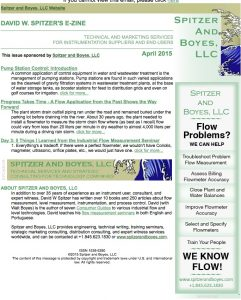# Level Gauge Performance (Part 2 of 3)A percentage of measured distance statement describes a parameter that is in error by a constant percentage of the actual distance measurement. In the measurement of a 1000 mm high vessel (100% level located 100 mm below the sensor) measured with an accuracy of 1 percent of measured distance, the absolute error can be calculated as:

An absolute (fixed) distance error statement describes an error that is constant. This error is independent of the calibration range and the actual level in the vessel. For example, the stated accuracy of a level measurement system in a 1000 mm high vessel (100% level located 100 mm below the sensor) might be ±10 mm. The absolute error at different levels is:

Level Absolute Error (1% of measured distance)
0% (empty) 1% of 1100 = 11.0mm
25% 1% of 850 = 8.5mm
50% 1% of 600 = 6.0mm
75% 1% of 350 = 3.5mm
100% (full) 1% of 100 = 1.0mm

A percentage of set span statement describes the error in terms of the full scale range. For example, the stated accuracy of a level measurement system in a 1000 mm high vessel (100% level located 100 mm below the sensor) might be ±1 percent of set span. The set span is 1100-100 or 1000mm, so the absolute error at different levels is:

Level Absolute Error (1% of set span)
0% (empty) 1% of 1000 = 10mm
25% 1% of 1000 = 10mm
50% 1% of 1000 = 10mm
75% 1% of 1000 = 10mm
100% (full) 1% of 1000 = 10mm

A percentage of maximum span statement describes the error in terms of the maximum sensor distance minus the blocking distance. For example, the stated accuracy of a level measurement system with a sensor that can measure from 400 mm to 8000 mm might be ±1 percent of the maximum span. The maximum span is 8000-400 or 7600 mm, so the absolute error at different levels is:

Level Absolute Error (1% of maximum span)
0% (empty) 1% of 7600 = 76mm
25% 1% of 7600 = 76mm
50% 1% of 7600 = 76mm
75% 1% of 7600 = 76mm
100% (full) 1% of 7600 = 76mm

In order to fairly compare performance, the same type of accuracy statement should be used for each level measurement system. For level measurement, the best measure of performance is usually the absolute (fixed) level error statement because it quantifies the amount of error expected to be present. Therefore, in most cases, statements should be expressed or converted to an absolute (fixed) level error statement before using the information for comparison purposes.

Note the significant variation in absolute errors associated with the different error statements above.

The preponderance of error statements used by suppliers will be discussed in Part 3.